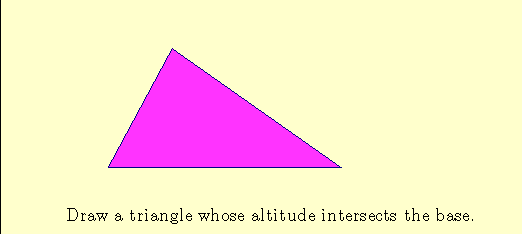Area of a Triangle  1.

The formula for the area of a triangle is A = (1/2)bh  where b is the base and h is the height.The justification above  used the fact that we drew the triangle so that the "highest point" was over the "base".  This holds, in particular, for any right triangle with any side viewed as the base.  If the altitude does not intersect the base, we can show that the result still holds by representing the area of the triangle as the difference of areas of two right triangles, as shown below.Animations by Bettina Richmond and Tom Richmond.
Tom.Richmond@wku.edu
Updated May 22, 2008.